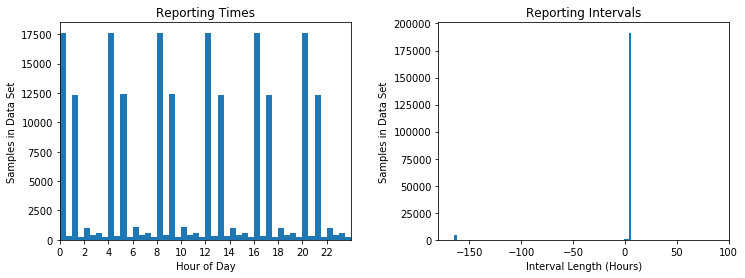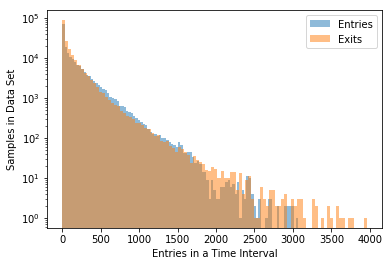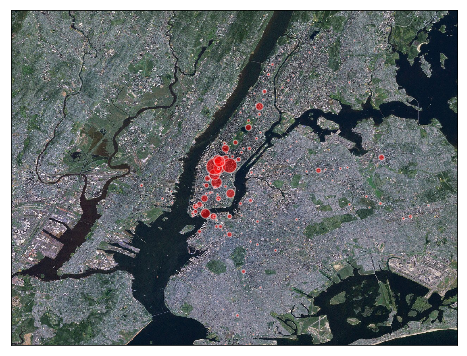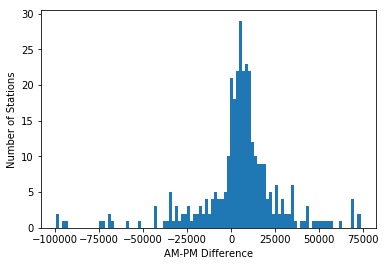# MTA Turnstile Data¶

The Metropolitan Transportation Authority (MTA) operates the New York City Subway. On their website, the MTA publishes data from the turnstiles in its subway stations. For each turnstile, passenger entries into and exits out of the subway station are logged accumulatively for four-hour periods: each turnstile has an entry and an exit counter and the data essentially provide the counter values every four hours.

In this notebook, we will first explore and prepare the turnstile data. Then we will determine the busiest stations and characterize stations as commuter origins or commuter destinations. Thereafter, we will explore the evolution of ridership over the course of the day and the year. Finally, we will build a linear regression model that reproduces the daily ridership.

In :
from IPython.core.display import display, HTML
display(HTML("<style>.container { width:60% !important; }</style>"))


First, some imports:

In :
import pandas as pd
import numpy as np
import matplotlib.pyplot as plt
from matplotlib import gridspec
from mpl_toolkits.basemap import Basemap


# Data Exploration and Preparation ¶

The data comes in indiviual files containing the turnstile data of one week. For now, we will explore one exemplary file from the week preceding 3/24/18.

In :
data = pd.read_csv('turnstile_180324.txt')
# Fixes read-in problem of last column name:
data = data.rename(columns = {data.columns[-1]:'EXITS'})
# Remove irrelevant columns
data = data.drop(['DIVISION','DESC','LINENAME'], axis=1)

In :
data.shape

Out:
197149

There are 197,149 samples in this data set.

In :
data.head()

Out:
C/A UNIT SCP STATION DATE TIME ENTRIES EXITS
0 A002 R051 02-00-00 59 ST 03/17/2018 00:00:00 6552626 2219139
1 A002 R051 02-00-00 59 ST 03/17/2018 04:00:00 6552626 2219140
2 A002 R051 02-00-00 59 ST 03/17/2018 08:00:00 6552626 2219140
3 A002 R051 02-00-00 59 ST 03/17/2018 12:00:00 6552626 2219140
4 A002 R051 02-00-00 59 ST 03/17/2018 16:00:00 6552626 2219140

The columns C/A, UNIT, and SCP denote identifiers for a specific turnstile. STATION is the name of the subway station. ENTRIES and EXITS are the accumulated counter values of the turnstile (not the number of entries in the given time interval).

We will create additional columns to make data evaluation easier:

In :
# Create timestamp column
data['timestamp'] = pd.to_datetime(data['DATE'] + ' ' + data['TIME'])

# Create column with length of interval since last data entry
data['interval'] = data['timestamp'] - data['timestamp'].shift(1)

# Calculate number of entries/exits in the preceding interval
data['ENTRY_DIFF'] = data['ENTRIES'] - data['ENTRIES'].shift(1)
data['EXIT_DIFF'] = data['EXITS'] - data['EXITS'].shift(1)


Out:
C/A UNIT SCP STATION DATE TIME ENTRIES EXITS timestamp interval ENTRY_DIFF EXIT_DIFF
0 A002 R051 02-00-00 59 ST 03/17/2018 00:00:00 6552626 2219139 2018-03-17 00:00:00 NaT NaN NaN
1 A002 R051 02-00-00 59 ST 03/17/2018 04:00:00 6552626 2219140 2018-03-17 04:00:00 04:00:00 0.0 1.0
2 A002 R051 02-00-00 59 ST 03/17/2018 08:00:00 6552626 2219140 2018-03-17 08:00:00 04:00:00 0.0 0.0
3 A002 R051 02-00-00 59 ST 03/17/2018 12:00:00 6552626 2219140 2018-03-17 12:00:00 04:00:00 0.0 0.0
4 A002 R051 02-00-00 59 ST 03/17/2018 16:00:00 6552626 2219140 2018-03-17 16:00:00 04:00:00 0.0 0.0

In order to better understand the structure of the data set, we can look at histograms for the time at which turnstile counter data are reported and the length of the reporting intervals.

In :
fig, ax = plt.subplots(1,2, figsize=(12,4), gridspec_kw={'wspace': 0.3})

#Plot histogram of times
ax.hist(data['TIME'].apply(pd.Timedelta) / pd.Timedelta('1 hour'),
bins=48)
ax.set_title('Reporting Times')
ax.set_xlabel('Hour of Day')
ax.set_xticks(range(24)[::2])
ax.set_xlim(0,24)
ax.set_ylabel('Samples in Data Set')

# Plot histogram of interval lengths
ax.hist(data['interval'].dropna() / pd.Timedelta('1 hour'), bins=100)
ax.set_title('Reporting Intervals')
ax.set_xlabel('Interval Length (Hours)')
ax.set_ylabel('Samples in Data Set')
plt.show()Most reporting times come in four-hour intervals, but at two separate time sequences: a little more than half the reporting times are at 0, 4, 8, 12, 16, and 20 hours (using the 24-hour format) and a large amount of the rest are reported at 1, 5, 9, 13, 17, and 21 hours.

There are a few samples with other reporting times but we will not take those into account. The small amount of reporting intervals of -168 hours are an artefact stemming from the time difference between the last sample of one turnstile and the first of the next turnstile. We will also eliminate those samples.

In the following, we remove:

• The first sample for each turnstile (we can't calculate the difference to the previous one)
• Samples with a negative number of entries or exits per interval
• Samples with more than 4000 entries/exits per interval (corresponds to 1 entry/exit in every 4 seconds, which is the highest frequency (aside from artifacts) found in the data)
• Samples with interval lengths that are not around 4 hours
In :
# Remove first entry of each turnstile
data['NEW_SCP'] = ~ (data['SCP'] == data['SCP'].shift(1))
data = data[ (data['NEW_SCP']==False)
# Remove negative no. of entries/exits:
& (data['ENTRY_DIFF']>=0) & (data['EXIT_DIFF']>=0) # only positive entries/exits
# Remove entry/exit rates that are too high
& (abs(data['ENTRY_DIFF'])<4000) & (abs(data['EXIT_DIFF'])<4000)
# Remove intervals that are not around 4 hours long
& (data['interval']/pd.Timedelta('1 hour')>3.9) & (data['interval']/pd.Timedelta('1 hour')<4.5)
].drop('NEW_SCP', axis=1)

In :
data.shape

Out:
189301

After eliminating these anomalous samples, we are still left with 189,301 which is about 96% of the original samples.

Of the remaining, realistic entry/exit data, we can plot a histogram of the number of samples with a certain entry/exit frequency:

In :
plt.hist(data['ENTRY_DIFF'], bins=100, alpha=0.5, label='Entries')
plt.hist(data['EXIT_DIFF'], bins=100, alpha=0.5, label='Exits')
plt.yscale('log', nonposy='clip')
plt.xlabel('Entries in a Time Interval')
plt.ylabel('Samples in Data Set')
plt.legend()
plt.show()# Busiest Stations¶

We now want to find out what the busiest stations are. For this purpose we will look at both entries and exits at any given station.

In :
# Group data by station
total_riders = data.groupby(['STATION'], as_index=False) \
[['ENTRY_DIFF','EXIT_DIFF']].sum()
# Columns for sum and difference of entries and exits
total_riders['TOTAL_DIFF'] = \
total_riders['ENTRY_DIFF'] + total_riders['EXIT_DIFF']
total_riders['ENTRY_EXIT_DEFICIT'] = \
total_riders['ENTRY_DIFF'] - total_riders['EXIT_DIFF']


The total_riders DataFrame contains the summed-up entries and exits for each station as well as their sum and difference. By sorting the DataFrame we can immediately tell the busiest stations. 34 St./Penn Station is the busiest with 1.7 million turnstile crossings in this week in March 2018.

In :
total_riders.sort_values(by=['TOTAL_DIFF'], ascending=False).head()

Out:
STATION ENTRY_DIFF EXIT_DIFF TOTAL_DIFF ENTRY_EXIT_DEFICIT
59 34 ST-PENN STA 893112.0 768412.0 1661524.0 124700.0
229 GRD CNTRL-42 ST 781770.0 698025.0 1479795.0 83745.0
57 34 ST-HERALD SQ 608904.0 572023.0 1180927.0 36881.0
45 23 ST 624999.0 459962.0 1084961.0 165037.0
14 14 ST-UNION SQ 580247.0 503932.0 1084179.0 76315.0

We now want to visualize the weekly ridership for each station on a map. The file stations_conversion.csv contains the geographic coordinates for each station. We will load these data and merge it with the total_riders DataFrame.

In :
stations = pd.read_csv('stations_conversion.csv')

In :
total_riders = pd.merge(total_riders, stations, on='STATION', how='inner')

Out:
STATION ENTRY_DIFF EXIT_DIFF TOTAL_DIFF ENTRY_EXIT_DEFICIT GTFS Latitude GTFS Longitude
0 1 AV 129461.0 143324.0 272785.0 -13863.0 40.730953 -73.981628
1 103 ST 173796.0 116370.0 290166.0 57426.0 40.795379 -73.959104
2 103 ST-CORONA 117369.0 84928.0 202297.0 32441.0 40.749865 -73.862700
3 104 ST 13785.0 6985.0 20770.0 6800.0 40.688445 -73.841006
4 110 ST 58883.0 48748.0 107631.0 10135.0 40.795020 -73.944250

Now we can plot the stations as markers on a Basemap map with the size of the markers corresponding to the total ridership of the station. The data are displayed on a satellite image retrieved using arcgisimage.

In :
plt.figure(figsize=(8, 8))

# Create map with basemap
m = Basemap(projection='cyl', resolution='i',
llcrnrlat = total_riders['GTFS Latitude'].min(),
llcrnrlon = total_riders['GTFS Longitude'].min(),
urcrnrlat = total_riders['GTFS Latitude'].max(),
urcrnrlon = total_riders['GTFS Longitude'].max())
m.arcgisimage(service='ESRI_Imagery_World_2D',
xpixels=1500, verbose=True)

# Draw stations with marker size according to ridership
for line in total_riders.iterrows():
x,y = m(line['GTFS Longitude'],line['GTFS Latitude'])
size = line['TOTAL_DIFF'] / 100000
plt.plot(x, y, 'o', markersize=size, color='red', alpha=0.5,
markeredgewidth=1, markeredgecolor='white')

plt.show()

http://server.arcgisonline.com/ArcGIS/rest/services/ESRI_Imagery_World_2D/MapServer/export?bbox=-74.19055,40.576127,-73.75540500000001,40.903125&bboxSR=4326&imageSR=4326&size=1500,1127&dpi=96&format=png32&f=imageFrom this map visualization we can tell that the busiest stations are located in Manhattan, specifically in Midtown.

# Commute¶

Using the MTA ridership data, we can learn about commute in New York City by identifying stations that people commute to (commuter destinations) and stations that people commute from (commuter origins).

We create two subsets of the data, one for the morning (data_am) and one for the evening (data_pm), and group them by station. We then merge them and create a new column am_pm_difference that reflects the difference in ridership in the morning and in the evening at a given station. More preciesly, a large am_pm_difference arises from more entries in the morning or exits in the evening, as well as less entries in the evening and less exits in the morning.

In :
# Morning data grouped by station
data_am = data[(data['TIME']=='08:00:00') | \
(data['TIME']=='09:00:00')]\
.groupby('STATION', as_index=False).sum()
# Evening data grouped by station
data_pm = data[(data['TIME']=='20:00:00') | \
(data['TIME']=='21:00:00')]\
.groupby('STATION', as_index=False).sum()

# Merge morning and evening data
commute = pd.merge(data_am, data_pm, on='STATION', suffixes=['am','pm'])

# Calculate difference
commute['am_pm_difference'] \
= commute['ENTRY_DIFFam'] + commute['EXIT_DIFFpm'] \
- commute['ENTRY_DIFFpm'] - commute['EXIT_DIFFam']


This difference (which is a measure for how many riders commute from this station) is plotted in the following histogram. It is immediately clear that more stations are commuter origins than commuter destinations.

In :
plt.hist(commute[abs(commute['am_pm_difference'])<100000]['am_pm_difference'], bins=100)
plt.xlabel('AM-PM Difference')
plt.ylabel('Number of Stations')
plt.show()We now want to plot these data for each station. First, we exclude anomalous values. Then, we merge the commute DataFrame with the station locations and plot them using Basemap.

In :
commute = commute[abs(commute['am_pm_difference'])<50000]

In :
commute = pd.merge(commute, stations, on='STATION', how='inner')

In :
fig = plt.figure(figsize=(8, 8))

# Create map with basemap
m = Basemap(projection='cyl', resolution='i',
llcrnrlat = commute['GTFS Latitude'].min(),
llcrnrlon = commute['GTFS Longitude'].min(),
urcrnrlat = commute['GTFS Latitude'].max(),
urcrnrlon = commute['GTFS Longitude'].max())
m.arcgisimage(service='ESRI_Imagery_World_2D',
xpixels = 1500, verbose= True)

# Draw stations with marker sizes according to AM-PM difference
for line in commute.iterrows():
x,y = m(line['GTFS Longitude'],line['GTFS Latitude'])
difference = line['am_pm_difference']
marker_size = 2000
if difference > 0: # Commuter origins
size = difference / marker_size
color = 'blue'
else:  # Commuter destinations
size = - difference/ marker_size
color = 'red'

plt.plot(x, y, 'o', markersize=size, color=color, alpha=0.5,
markeredgewidth=1, markeredgecolor='white')

plt.show()

http://server.arcgisonline.com/ArcGIS/rest/services/ESRI_Imagery_World_2D/MapServer/export?bbox=-74.073643,40.576127,-73.75540500000001,40.903125&bboxSR=4326&imageSR=4326&size=1500,1541&dpi=96&format=png32&f=image